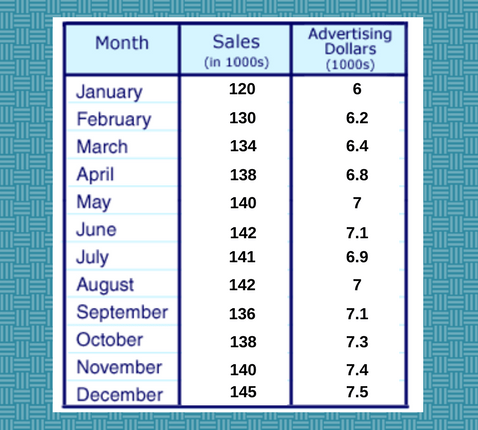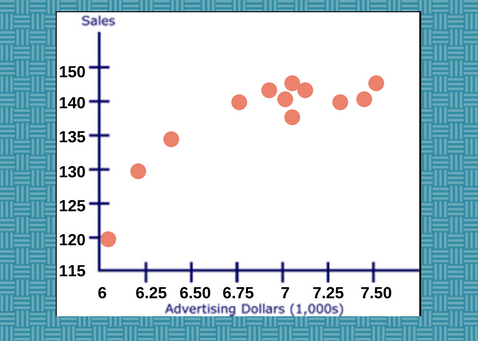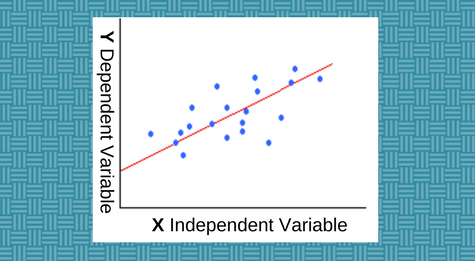# Linear Regression Models: Explained

Linear regression models are the most basic types of statistical techniques and widely used predictive analysis. They show a relationship between two variables with a linear algorithm and equation.

Linear regression modeling and formula have a range of applications in the business. For example, they are used to evaluate business trends and make forecasts and estimates. They can also be used to analyze the result of price changes on the consumer behavior. It can be given numerous examples.

On this page:

• What is linear regression all about?
• Simple linear regression models
• Multiple modeling
• Infographic in PDF for free Download

## What Is Linear Regression?

Simply, linear regression is a statistical method for studying relationships between an independent variable X and Y dependent variable.

To put it in other words, it is a mathematical modeling which allows you to make predictions and prognosis for the value of Y depending on the different values of X.

Just to note that
• the dependent variable is also known as a response variable
• independent variables are also known explanatory or predictor variables

In the reality, you can have only one independent variable X that affects the dependent variable Y. Or, you can have cases where there are many independent variables that affect Y.

Based on this approach, there are two main types of linear regression:

• Simple linear regression models
• Multiple linear regression models

### Simple VS Multiple Linear Regression Models

What is simple linear regression?

As you might guess, simple linear regression means there is only one independent variable X which changes result on different values for Y.

Its model/ formula is:

Y = Β0 + Β1X

Where:

X – the value of the independent variable,
Y – the value of the dependent variable.
Β0 – is a constant (shows the value of Y when the value of X=0)
Β1 – the regression coefficient (shows how much Y changes for each unit change in X)

When is simple linear regression modeling appropriate?

It is appropriate when the following conditions are satisfied:

First Condition:
• First, you need to be sure, the dependent variable has a linear relationship to the independent variable. How to check that? Make sure that the XY scatterplot is linear.

What is scatterplot? This is a graphic tool that displays the relationship between two variables. Actually, one of the basics steps in regression modeling is to plot your data on a scatter plot.

To make the things clear, let’s see an example:

The following table shows the monthly sales and advertising costs for last year by a business software company.In this case, your plot for monthly sales and advertising costs would be:Note:

The data for your independent and dependent variables must be from the same period of time.

Scatter plots are very effective and widely used in visually identifying relationships between different variables.

These relationships are expressed mathematically in terms of a correlation coefficient ( known also as a correlation). Correlations are indicators of the strength of the relationship between the independent and dependent variable. They can be in the range from –1 to +1.

A positive correlation means that if the independent variable gets bigger, the dependent variable tends to get bigger. The larger the absolute value of a the correlation coefficient, the stronger the linear relationship.

In our above simple linear regression model formula, Β1 is the regression coefficient.

Second Condition:
• The second condition you need, a simple linear regression to be appropriate is: for each value of X, the likelihood distribution of Y has the same standard deviation σ.

When this condition is fulfilled, the variability of the residuals will be comparatively constant across all values of X. You can check this with the help of residual plot.

The residual plot is a graph that represents the residuals on the vertical axis and the independent variable on the horizontal axis. If the points are randomly dispersed around the horizontal axis, linear regression models are appropriate for the data. If not, non-linear models are more appropriate.

Here is an example of a residual plot:How to Define a Regression Line?

Commonly, with the help of a software tool (e.g., Excel) or a special graphing calculator – to find b0 and b1. It is very easy with the calculator. You just enter the values of X and Y into the calculator, and the tool resolves for each parameter.

What is Multiple Linear Regression?

Multiple linear regression model is the most popular type of linear regression analysis. It is used to show the relationship between one dependent variable and two or more independent variables.

In fact, everything you know about the simple linear regression modeling extends (with a slight modification) to the multiple linear regression models.

Let’s see the model.

The following formula is a multiple linear regression model.

Y = Β0 + Β1X1 + Β2X+…..ΒpXp

Where:

X, X1, Xp – the value of the independent variable,
Y – the value of the dependent variable.
Β0 – is a constant (shows the value of Y when the value of X=0)
Β1, Β2, Βp – the regression coefficient (shows how much Y changes for each unit change in X)

This model is linear because it is linear in the parameters Β0, Β1, Β2 and … Βp

Conclusion

Nowadays, businesses accumulate all types of data such sales performance data, net and gross profit, competition information, customer profiles and other information needed for business and market analysis.

Linear regression is a statistical method that has a wide variety of applications in the business world. Simple and multiple linear regression models can be used by companies to evaluate trends and make forecasts.

It can be used also to analyze the result of pricing on consumer behavior and buying intentions, to assess different types of risks and etc.

So as you see, linear regression is a powerful statistical modeling that can be used to gain insights on consumer behavior and to understand factors that influence business profitability and effectiveness.

Download the following infographic in PDF for FREE